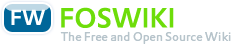# Course Digital Systems

Responsible: Prof.Dr.Thieling

## Course

### Meets requirements of following modules(MID)

• in active programs

### Course Organization

Version
created 2013-07-24
VID 1
valid from WS 2012/13
valid to
Course identifiers
Long name Digital Systems
CID F07_DT
CEID (exam identifier)

Contact hours per week (SWS)
Lecture 2
Exercise (unsplit)
Exercise (split) 1
Lab 1
Project
Seminar
Tutorial(voluntary)
Total contact hours
Lecture 30
Exercise (unsplit)
Exercise (split) 15
Lab 15
Project
Seminar
Tutorial (voluntary)
Max. capacity
Exercise (unsplit)
Exercise (split) 30
Lab 15
Project
Seminar

Total effort (hours): 150

• Deutsch

• Bachelor

#### Prerequisites

• none

• Urbanski K., Woitowikz R.: Digitaltechnik, 4. Auflage Springer 2004
• Beuth K.: Elektronik Bd. 4 Digitaltechnik, Vogel Verlag 2001
• Lipp H.M.: Grundlagen der Digitaltechnik, 4. Auflage Oldenbourg 2002

#### Instructors

• Prof. Dr. Thieling
• Prof. Dr. Hartung

#### Supporting Scientific Staff

• Dipl.-Ing. Peter Pohlig

Digital Systems

### Assessment

Type
wE normal case (except on small numbers of assessments: oE

Total effort [hours]
wE 10

Frequency: 3/Jahr

## Course components

### Lecture/Exercise

#### Objectives

##### Contents
• boolean algebra
• basic functions
• axioms and laws
• disjunctive normal form, minterms
• conjunctive normal form, maxterms
• systematic simplification
• boolean network
• logic gates, tri-state buffer
• description forms
• boolean equation
• table
• KV diagram
• schematic
• transformations between the forms of description
• analysis
• synthesis (including transfer from text to problem solution)
• don't-care conditions
• typical networks
• decoder
• multiplexer
• demultiplexer
• hazards
• static hazard
• function hazard
• dynamic hazard
• avoid hazards
• codes
• weighted codes
• dual
• octal
• change of basis
• BCD codes and their applications
• gray codes and their applications
• properties
• redundancy
• hamming distance
• cyclicness
• parity ans block codes
• number representation in computer systems
• two's complement
• fixed point representation
• floating point representation
• ASCII-code
• feedback networks
• flip-flops and latches
• RS
• D
• JK
• asynchronous control
• clock state control
• edge triggered
• registers
• shift register
• parallel-serial conversion
• seria-parallell conversion
• practice-oriented specifications
• setup time
• hold time
• minimum puls width
• synchronous counters
• the basic idea
• construction using D flip-flops
• analysis
• synthesis
• specification using VHDL
• refer VHDL
• finite state machines
• description of state machines using state transition diagrams (Moore and Mealy)
• design of state machines as a problem-solving
• implementation using D-flops
• Implementation using VHDL
• state transition diagrams
• modeling according to Moore
• modeling according to Mealy
• conversion between Moore and Mealy
• characteristics (determinism, completeness)
• VHDL
• specification of boolean networks
• structure of a VHDL program (entity, port, architecture, signals, in, out)
• signals (type stdlogic: 1, 0, Tri-State, Don't-Care)
• signal assignment (direct implementation of boolean functions)
• conditional signal assignment (direct conversion of tables)
• vectors of signals
• integer data type and conversion from/to signal vectors
• design entry VHDL
• specification of counters and finite state machines
• processes and sequential instructions (process, variable, if, case, event, type)
• implementation of regular counter in VHDL
• implementation of finite state machines in VHDL
• programmable logic devices
• structure
• the basic idea
• technology
• CPLD versus FPGA
• design tool
• scematic design entry
• basic library (gates, in, ou, buffer, mux, decoder, flip-flops)
• buses
• hierarchical schematics
• VHDL design entry
• detail refer VHL
• synthesis
• simulation
• without propagation delay
• with propagation delay
• structure and mode of operation of a simple computer
• structure of a Von Neumann computer (registers, arithmetic logic unit, control unit, memory, buses)
• mode of operation (program execution based on register transfers)
• concretization of mode of operation (a minimal simulated Von Neumann computer)
• programming the minimal computer in assembler (simple loops, different addressing modes)

##### Acquired Skills
• specifying system behavior (derived from text documents)
• development of problem solutions that can be implemented with boolean networks
• interpretation and convertion of codes
• development of problem solutions that can be implemented with synchronous counters
• development of problem solutions that can be implemented with finite state machines
• explain the operation of a Von Neumann computer

• none

### Lab

#### Objectives

##### Acquired Skills
• development od digital systems
• explain the system behavior of a Von Neumann computer
• implement subsystems of a Von Neumann computer
• implementation of C-code sequences using assembler

##### Operational Competences
• manage complex tasks as a small team
• develop problem solutionsDeutsch English